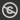Science, Maths & Technology
Free course

# Second-order differential equations## Course reviews

This free course is concerned with second-order differential equations. Section 1 introduces some basic principles and terminology. Sections 2 and 3 give methods for finding the general solutions to one broad class of differential equations, that is, linear constant-coefficient second-order differential equations. Section 2 covers homogeneous equations and Section 3 covers inhomogeneous equations. Section 4 explains how extra information can be used to help to select particular solutions that are appropriate in given situations. Section 5 looks at the phenomenon of resonance to gain insights into the solutions of inhomogeneous linear constant-coefficient second-order differential equations.

## Course learning outcomes

After studying this course, you should be able to:

• understand the key role of the principle of superposition in the solution of linear constant-coefficient second-order differential equations
• obtain the general solution of a homogeneous linear constant-coefficient second-order differential equation using the solutions of its auxiliary equation
• use the method of undetermined coefficients to find a particular integral for an inhomogeneous linear constant-coefficient second-order differential equation with certain simple forms of right-hand-side function
• obtain the general solution of an inhomogeneous linear constant-coefficient second-order differential equation by combining its complementary function with a particular integral
• use the general solution together with a pair of initial or boundary conditions to obtain, when possible, a particular solution of a linear constant-coefficient second-order differential equation.

First Published: 13/12/2017

Updated: 29/04/2019

Skip Rate and Review